top of page

## 10  Noise Coupling

Noise coupling is one of the essential and constantly recurring topics in EMC. An EMC design engineer must consider any noise coupling paths constantly during the design and when troubleshooting.

Couplin Paths

Coupling Paths.

One of the most important concepts to understand in EMC is the concept of coupling
paths. To start off, let’s see what parts are involved when Electromagnetic Interference (EMI) happens and why focusing on coupling paths is so important [10.1]:

1. Source. In the real world, there are sources of unwanted electric or electromagnetic noise. For EMC immunity tests, the noise sources are well defined with the goal that these sources should be as close as possible to the real world (e.g., ESD generators, burst generators, surge generators, and antennas).

2. Coupling path. The noise needs a path from the source to the victim. This path is called the coupling path or coupling channel.

3. Victim. The victim is the receiver or receptor of the noise, which could cause
interference.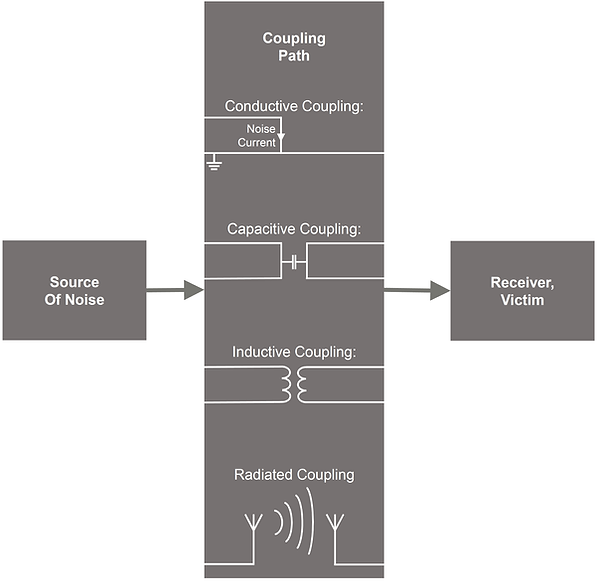The figure above shows us that if there are issues with EMI and EMC, one has the following three options to overcome these issues [10.1]:

1. Reduce the noise level of the noise source.

• Emission testing. When testing for radiated emissions of a product (meaning: the EUT is the source of noise), the noise level can be reduced by adding filters and shields to the EUT, controlling the signal transients (rise-/fall-time), or performing a complete redesign (e.g., change from single-ended to differential signaling).

• Immunity testing. The applicable EMC product standard specifies the noise level of the noise source for EMC immunity tests. Therefore, there is no option to reduce the noise level for EMC immunity tests.

2. Make changes to the coupling path. The coupling path is where an EMC engineer usually has to focus on if a product fails an EMC test. With well-considered changes to the coupling path, emissions can be reduced and immunity increased (e.g., improving ground connections, adding filters and shields to cables and PCBs).

3. Increase the victim’s immunity level with software or firmware. Possible software features which can increase a product’s EMC immunity are: digital filters (e.g., median), spike removers (remove spikes in sensor signals), and sanity checks.

There are different kinds of coupling paths:

1. Conductive coupling (common impedance coupling)

2. Capacitive coupling (electric field coupling)

3. Inductive coupling (magnetic field coupling)

4. Radiated coupling (electromagnetic field coupling)

Conductive Coupling. Conductive coupling can occur when two or more circuits share a common path or common conductor and, therefore: a common impedance Zcom [W]. This shared path leads typically to ground or another reference plane.

Why could a common conductor path lead to EMC problems or a failed EMC test case? Here is an example: If one of the two circuits, from the figure below, experiences an ESD, burst, or surge pulse, a high current may flow for a short time through a common conductor segment and introduces a noise voltage to the other circuit, which may lead to a malfunction. This is the reason why it is crucial having low-impedance earth/ground planes. A low-impedance ground plane helps to minimize the conductive coupling. In addition, a common path often leads to bad signal integrity as well.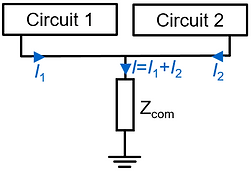Capacitive Coupling. Capacitive coupling can occur if there is a coupling capacitance C  [F] between two circuits (see figure below [10.1]). The field of concern for capacitive coupling is the electric E-field. Thus, capacitive coupling is a near-field coupling, which means that the noise source and the victim, which receives the noise, are closely located to each other.

It is assumed that the capacitive coupling discussed in this section is a weak coupling. That means that the noise coupling is a one-way effect from the noise source to the victim circuit (negligible back reaction from victim to source).For a very large R [Ω], the conductive noise coupling is independent of the frequency ω [rad/sec] and the noise voltage Vn [V] is of larger amplitude than when R [Ω] is small. The frequency response in the figure below confirms that [10.1].Possible measures against a capacitive coupling (assuming that the voltage level V1 [V] of the noise generator and the victim circuit load R [Ω] cannot be changed):

• Reduce transient of noise source. Because the noise current through the stray capacitance in(t)=C12dv1/dt is directly proportional to the voltage transient dv1=dt [V/s] of the noise source, a reduction of the voltage transient in the noise source helps minimize the capacitive coupling. Note: this measure does not help in the case of very high impedance victim circuits, because in this case, the coupling does not depend on the frequency ω [rad/sec].

• Reduce coupling capacitance. No coupling capacitance means no capacitive coupling. In practice, the coupling capacitance cannot be removed completely, but it can be minimized with several measures:

• Shielding. Adding a shield around the noise source or the victim reduces the coupling capacitance to a minimum (given that the shield does cover the complete noise source and/or victim). Shielding against capacitive coupling is already achieved by connecting only one end of the shield to ground [10.2].

• Spatial separation. If possible, the source of noise and the victim circuit could be spatially separated from one another.

• Change conductor orientation. Let us say the noise source circuit and the victim circuit have conductors parallel to each other. If feasible, a change of the orientation to an angle of 90° between the wires would reduce the coupling capacitance.

• Filtering. With a filter added to the victim’s circuit, the coupled noise could be reduced. For high-impedance victim circuits, adding a filter capacitor parallel to R [Ω] would increase C2G [F] and therefore help to reduce |Vn| [V].

Inductive Coupling. Inductive coupling can occur if there is a mutual inductance M [H] between two or more circuits. The field of concern for inductive coupling is the magnetic H-field. Thus, inductive coupling is a near-field coupling, which means that the noise source and the victim, which receives the noise, are closely located to each other.

It is assumed that the inductive coupling discussed in this section is a weak coupling. That means that the noise coupling is a one-way effect from the noise source to the victim circuit (negligible back reaction from victim to source).Differential-Mode vs. Common-Mode

Some possible measures against an inductive coupling (assuming that the amplitude of the noise current I1 [A] cannot be changed):

• Reduce current loop area. The most effective and best way to overcome inductive coupling is to reduce the current loop area A [m2] of the victim’s circuit. Therefore, always take care that the current loop of sensitive circuits is kept as small as possible.

• Spatial separation. The physical separation of the noise source and the victim circuit leads to a reduced magnetic flux density B [T] (when it reaches the victim current loop).

• Shielding. A cable shield for eliminating inductive coupling must be grounded at both ends (a non-magnetic shield grounded only on one end does not affect a magnetic near-field coupling) [10.2]). Magnetic fields with low-frequency are best shielded with magnetic conductive shielding material (µr'<<1).

• Change circuit orientation. The magnetic flux density is a vector field 􀀀B [T], which means it has a defined direction. Therefore, a possible option to reduce the noise due to inductive coupling is to change the orientation of the victim's current loop area so that the term cos(phi) gets zero.

Electromagnetic Coupling. Electromagnetic coupling is a far-field coupling. This means that the noise source and the victim are located far from each other compared to the wavelength λ [m]. The field of concern for radiated coupling is the electromagnetic field, where the H-field and the E-field travel perpendicular to each other, and their amplitudes both fall off with the factor 1/d, where d [m] is the distance to the radiating source.

The electromagnetic coupling also plays an important role during EMC tests. E.g., during the radiated immunity test according to IEC 61000-4-3, where the EUT is placed in the far-field of the radiating E-field test antenna (80MHz to 6GHz, typically d=3m). Moreover, during radiated emission testing according to CISPR32, where the EUT is the radiator and the receiving antenna is an E-field antenna placed in the far-field (30MHz to 6GHz, typically d=3m or d=10m).

Here are some typical options on how to reduce unwanted electromagnetic coupling:

• Filtering. Suppose a cable is identified as a transmitter or receiver of electromagnetic radiation. In that case, a clamp ferrite bead may reduce the unintended radiation and/or increase immunity (the clamp ferrite bead attenuates common-mode currents through the cable). Also, filter elements like capacitors placed on the PCB for every IO-line of a cable can help to filter high-frequency signals in either direction. For differential signal interfaces, common-mode chokes are also a good filter option.

• Shielding. Put a shield around the circuit, cable, or unit that unintentionally radiates or acts as a receiver of electromagnetic radiation.

• Grounding. If a metal chassis or a subsystem within the EUT is the source/sink of the electromagnetic radiation, the ground connection should be checked and improved (low-inductance ground connection). Poor grounding of PCBAs, cable shields, or metal structures often leads to unwanted electromagnetic coupling.

Differential-Mode vs. Common-Mode.

In EMC, a distinction is made between common-mode and differential-mode noise currents. Both noise currents could lead to unintended radiation. The figure below shows the difference between differential- and common-mode signals.

• Differential-mode voltage. The differential-mode voltage VDM [V] is defined as the difference of the voltage potentials to ground of conductor 1 and conductor 2: VDM=V1􀀀-V2.

• Common-mode voltage. In the case of a balanced transmission line (both conductors have equal impedances to ground), the common-mode voltage VCM [V] is 0.5 of the sum of the voltages of conductor 1 and conductor 2 to ground: VCM=(V1+V2)/2. However, in the case of an unbalanced transmission line, things are getting a little more complicated, and therefore, we have to define the imbalance factor h (see below).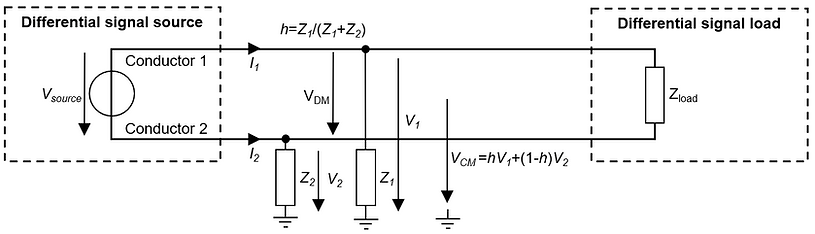The difference between differential-mode and common-mode current:

• Differential-mode current. A differential-mode current flows in different directions through a cable.

• Common-mode current. A common-mode current flows in the same direction - a common direction 􀀀- through a cable (see I1 and I2 in the figure above).

For a transmission line with two conductors, like shown in the figure above,􀀀 the imbalance factor h is defined as [10.3]:where, h is the imbalance factor or current divisor factor of a transmission line, Z1 [Ω] is the characteristic impedance of conductor 1 with respect to ground, Z2 [Ω] is the characteristic impedance of conductor 2 with respect to ground.
The imbalance factor h is used for calculating the common-mode voltage VCM [V] of any transmission line for a given differential-mode voltage VDM [V] [10.3]:where, VDM [V] is the differential-mode voltage between conductor 1 and 2, VCM [V] is the common-mode voltage to ground, V1 [V] is the voltage of conductor 1 with respect to ground, V2 = voltage of conductor 2 with respect to ground and h is the imbalance factor of the transmission line.

Differential-Mode Noise Sources

Differential-Mode Noise Sources.

Differential-mode noise sources cannot be found on any bill of material (BOM) or any schematic. These noise sources are unwanted and unintended.A not conclusive list of possible differential-mode interference noise sources is given here and then shortly explained in the following sections:

• Conductive coupling to differential-mode noise

• Capacitive coupling to differential-mode noise

• Inductive coupling to differential-mode noise

Conductive coupling to differential-mode noise.

Two or more circuits share a common current path (e.g., a common return current path), and the voltage drop of one of these circuits introduces a differential-mode noise voltage VnDM [V] in another circuit(s) and vice versa (see figure below).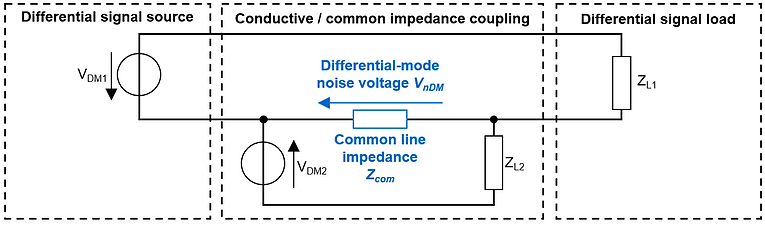Capacitive Coupling To Differential-Mode Noise.

Assuming a noise current InDM [A] is coupled capacitively to one of the two lines of a differential signal. This noise current causes differential-mode noise voltage VnDM [V] and could therefore lead to problematic interference on that circuit or unintended RF emissions (see figure below).Inductive Coupling To Differential-Mode Noise.

Let’s imagine a magnetic field that induces a noise voltage Vn [V] to a differential signal current loop which leads to a differential-mode noise current In [A] in that circuit (see figure below). This induced noise voltage and the noise current could lead to e.g. problematic interference on that circuit or unintended RF emissions.Common-Mode Noise Sources.

Common-mode noise sources cannot be found on any bill of material (BOM) or any schematic. These noise sources are unwanted and unintended. Common-mode currents are the number one source of unintentional radiated emissions.

Common-Mode Noise SourceA not conclusive list of possible common-mode interference noise sources is
given here and then shortly explained in the following sections:

• Capacitive coupling to common-mode noise

• Electromagnetic coupling to common-mode noise

• Reference point noise to common-mode noise

• Differential-to-common-mode conversion

Capacitive Coupling To Common-Mode Noise.

Let us imagine that noise currents are coupled capacitively (and equally) onto two differential signal lines, which form a current loop. As a consequence, the coupled common-mode current InCM [A] could lead to radiated or conducted emission issues. The figure below shows a simplified setup of how electric near-field coupling could cause common-mode noise.Electromagnetic Coupling To Common-Mode Noise.

A cable or a part of an electronic circuit acts as an antenna and receives electromagnetic radiation, e.g., from radio stations or smartphones, etc. The receiving structure is in the far-field of the transmitter. With this coupling, an unintended noise current is brought to an electrical circuit and could lead to interference.Reference Point Noise To Common-Mode Noise.

A noisy reference point (ground) or a voltage potential difference of spatially divided circuits and systems could cause a common-mode noise current InCM [A] and, therefore, high radiated emissions. The figure below shows in a simplified form a setup with a noisy reference point. The noise source voltage VnCM [V] itself could be caused by a current-driven differential-to-common-mode conversion [10.5].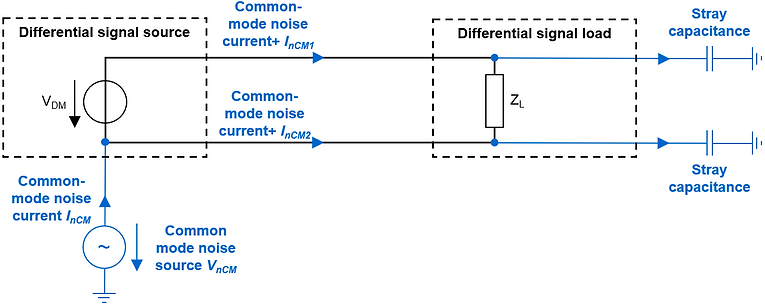Differential-To-Common-Mode Conversion.

Any change in electrical balance to ground of a transmission line converts some power of the differential-mode signal into a common-mode signal [10.3]. This differential-mode to common-mode conversion happens if 􀀀 and only if 􀀀 there is a change in balance of the transmission line [10.6]. Because this circumstance often causes high radiated emissions and failed EMC tests, the differential-to-common-mode conversion will be analyzed in the following by applying the Imbalance Difference Modeling method (IDM [10.3][10.7]).

Consider a transmission line that experiences a sudden change in the impedance of its lines to ground. This is typically the case when the transmission line leads from a PCB to a cable: in the connector, the individual conductors of the transmission line experience a sudden change in the characteristic impedance to ground (change in balance or imbalance). Let us look at the figure below, where a differential-mode signal experiences a sudden change in impedance to ground (at the imbalance interface). This sudden change leads to a common-mode voltage VAM [V] and common-mode current IAM [A], which we call antenna-mode voltage and antenna-mode current because they could lead to unintended radiation.We defined the imbalance factor h in an equation ablove. On the left side of the imbalance interface, conductors 1 and 2 have an imbalance factor of hL=Z1L/(Z1L+Z2L), and on the right side of the imbalance interface, the conductors have an imbalance factor of hR=Z1R/(Z1R+Z2R). The common-mode voltage VAM [V] and current IAM [A] that are developed due to the imbalance change are given as [10.3][10.7]:where, VDM [V] is the differential-mode voltage at the imbalance interface, VAM [V] is the common-mode voltage developed at the imbalance interface, Δh=hL􀀀-hR is the change of imbalance factor h at the imbalance interface, IAM [A] is the common-mode current through conductor 1 and 2 and ZAM [Ω] is the input impedance of antenna that is formed by conductors 1 and 2.
Imbalance difference modeling (IDM) is a powerful method for quickly calculating the worst-case common-mode voltage driving a cable (as an antenna). As a worst-case, it can be assumed an imbalance factor change of Δh=0.5 (perfectly balanced to completely unbalanced and vice versa), which means that the developed common-mode voltage is half the differential-mode amplitude:In EMC, the theoretical maximum field strength Emax [V/m] for a given setup at distance d [m] in the far-field is of primary interest. Therefore, here is a way for a worst-case estimation of the radiated emissions of a digital signal due to transmission line imbalance (e.g., change from an unbalanced microstrip line to a balanced ribbon-cable):

1. Differential-mode voltage VDM. Use the Fourier analysis to determine the RMS amplitude VDM [V] of the sinusoidal harmonics of the differential-mode signal.
Assumption: ideal square wave with 0sec rise-/fall-time and 50% pulse duration, we can approximate the RMS VDM [V] as:where, Vn,RMS [V] is the RMS value of the n-th harmonics sine wave, Vpp [V] is the peak-to-peak value of the ideal square wave, n is the number of the odd harmonic [1, 3, 5, ...], even harmonics have an amplitude of 0V for an ideal square wave signal.

2. Common-mode voltage VAM. Calculate the assumed worst-case common-mode RMS voltage VAM [V] developed at the transmission line imbalance change for the harmonics of interest [10.3]:3. Worst-case radiated emissions EAM,max. The approximate maximum E-Field from a common-mode voltage VCM=VAM [V] driving a cable at resonance [10.1]:where, Emax [V/m] is the maximum RMS E-field at distance d [m] for matched impedances, matched polarization, line-of-sight path and a resonant structure (that is resonant at the frequency of VCM) driven by VCM, VCM [V] is the RMS common-mode voltage that drives the resonant structure and d [m] is the distance from the antenna, where Emax [V/m] is measured.

bottom of page